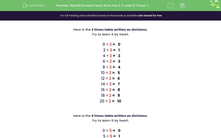# Recall Division Facts from the 2, 5 and 10 Times Tables

In this worksheet, students will recall division facts from the 2, 5 and 10 times tables.Key stage:  KS 1

Curriculum topic:   Number: Multiplication and Division

Curriculum subtopic:   Use Multiplication/Division Facts (2, 5, 10)

Difficulty level:#### Worksheet Overview

This activity will give you loads of practice at division - lucky you!Say you have 20 cakes and you want to share them onto 2 plates. If we want to divide 20 into 2 groups, we have to find how many 2s there are in 20. We count up to 20 in 2s, counting how many 2s it takes.

2, 4, 6, 8, 10, 12, 14, 16, 18, 20

How many 2s did you add on to get to 20?

It was 10, so 20 ÷ 2 = 10

This means that you can put 10 cakes on each plate!

Clearly, if you know that 10 x 2 = 20, you have a head start with the matching division calculation of 20 ÷  2

Here is the 2 times table written as divisions.

Try to learn it by heart.

0 ÷ 2 =   0

2 ÷ 2 =   1

4 ÷ 2 =   2

6 ÷ 2 =   3

8 ÷ 2 =   4

10 ÷ 2 =   5

12 ÷ 2 =   6

14 ÷ 2 =   7

16 ÷ 2 =   8

18 ÷ 2 =   9

20 ÷ 2 =   10How about if you wanted to share or divide 30 into 5 groups, for example, 30 strawberries to share between 5 people.

If you know your 5 times table, you will know that 6 x 5 = 30 and so the answer will be 6.

If you're not so confident with your tables, you could work out how many 5s there are in 30 by counting up in 5s. 5, 10, 15, 20, 25, 30

How many 5s did it take to get to 30?

It took 6 so we know that 30 ÷ 5 = 6

Here is the 5 times table written as divisions.

Try to learn it by heart.

0 ÷ 5 =   0

5 ÷ 5 =   1

10 ÷ 5 =   2

15 ÷ 5 =   3

20 ÷ 5 =   4

25 ÷ 5 =   5

30 ÷ 5 =   6

35 ÷ 5 =   7

40 ÷ 5 =   8

45 ÷ 5 =   9

50 ÷ 5 =   10

What about the 10 times table? If we want to find out 80 ÷ 10, we have to work out how many 10s there are in 80.

We can count up in 10s: 10, 20, 30, 40, 50, 60, 70, 80

How many 10s did it take? it took 8, so 80 ÷ 10 = 8

Here is the 10 times table written as divisions.

Try to learn it by heart.

0 ÷ 10 =   0

10 ÷ 10 =   1

20 ÷ 10 =   2

30 ÷ 10 =   3

40 ÷ 10 =   4

50 ÷ 10 =   5

60 ÷ 10 =   6

70 ÷ 10 =   7

80 ÷ 10 =   8

90 ÷ 10 =   9

100 ÷ 10 =   10

Now let's have a go at some questions.### What is EdPlace?

We're your National Curriculum aligned online education content provider helping each child succeed in English, maths and science from year 1 to GCSE. With an EdPlace account you’ll be able to track and measure progress, helping each child achieve their best. We build confidence and attainment by personalising each child’s learning at a level that suits them.

Get started